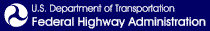Skip to content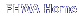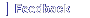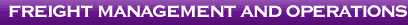# Bridge Formula Weights

Printable version [PDF 3.2 MB]
Contact Information: Operations Feedback at OperationsFeedback@dot.govU.S. Department of Transportation
Office of Operations
1200 New Jersey Avenue, SE
Washington, DC 20590
ops.fhwa.dot.gov

August 2019
FHWA-HOP-19-028

## Note

The Federal Highway Administration (FHWA) revises its guidance pamphlet Federal Bridge Formula Weights (May 2015). Previous editions of this guidance pamphlet are superseded and no longer valid. This guidance paraphrases provisions of 23 U.S.C. § 127 and 23 C.F.R. § 658 for purposes of illustration only. In the event of a dispute, the statute and regulation take precedence with respect to maximum allowable Federal Bridge Formula weights.

## Bridge Formula Weights

With a few exceptions noted in this pamphlet, the Bridge Formula establishes the maximum weight any set of axles on a motor vehicle may carry on the Interstate highway system. This pamphlet describes the Bridge Formula, why it was established, and how it is used.

## What Is It?

Congress enacted the Bridge Formula in 1975 to limit the weight-to-length ratio of a vehicle crossing a bridge. This is accomplished either by spreading weight over additional axles or by increasing the distance between axles.

Compliance with Bridge Formula weight limits is determined by using the following formula: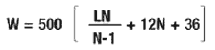W = the overall gross weight on any group of two or more consecutive axles to the nearest 500 pounds.

L = the distance in feet between the outer axles of any group of two or more consecutive axles.

N = the number of axles in the group under consideration.

In addition to Bridge Formula weight limits, Federal law states that single axles are limited to 20,000 pounds, and axles spaced more than 40 inches and not more than 96 inches apart (tandem axles) are limited to 34,000 pounds. Gross vehicle weight is limited to 80,000 pounds (23 U.S.C. 127).

## Is the Formula Necessary?

Bridges on the Interstate System highways are designed to support a wide variety of vehicles and their expected loads. As trucks grew heavier in the 1950s and 1960, something had to be done to protect bridges. The solution was to link allowable weights to the number and spacing of axles.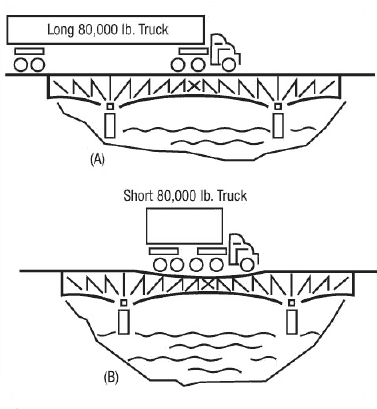Figure 1

Axle spacing is as important as axle weight in designing bridges. In Figure 1A, the stress on bridge members as a longer truck rolls across is much less than that caused by a short vehicle as shown in Figure 1B, even though both trucks have the same total weight and individual axle weights. The weight of the longer vehicle is spread out, while the shorter vehicle is concentrated on a smaller area.

## How Is the Formula Used?

The weight on various axle configurations must be checked to determine compliance with the Bridge Formula. Three definitions are needed to use the Bridge Formula correctly.

• Gross Weight—The weight of a vehicle or vehicle combination and any load thereon. The Federal gross weight limit on the Interstate System is 80,000 pounds unless the Bridge Formula dictates a lower weight limit.
• Single-Axle Weight—The total weight on one or more axles whose centers are spaced not more than 40 inches apart. The Federal single-axle weight limit on the Interstate System is 20,000 pounds.
• Tandem-Axle Weight—The total weight on two or more consecutive axles whose centers are spaced more than 40 inches apart but not more than 96 inches apart. The Federal tandem-axle weight limit on the Interstate System is 34,000 pounds.

Interstate System weight limits in some States may be higher than the figures noted above due to "grandfather" rights. When the Interstate System axle and gross weight limits were adopted in 1956, and amended in 1975, States were allowed to keep or "grandfather" weight limits that were higher.

Bridge Formula calculations yield a series of weights (Bridge Table, pages 5-6). It is important to note that the single-axle weight limit replaces the Bridge Formula weight limit on axles not more than 40 inches apart, and the tandem-axle weight limit replaces the Bridge Formula weight limit for axles over 40 but not more than 96 inches apart. At 97 inches apart, for example, two axles may carry 38,000 pounds (Figure 2A) and three axles may carry 42,000 pounds, as shown in Figure 2B.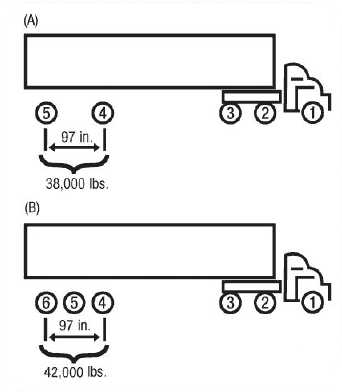Figure 2

Federal law states that any two or more consecutive axles may not exceed the weight computed by the Bridge Formula even though single axles, tandem axles, and gross weight are within legal limits. As a result, the axle group that includes the entire truck—sometimes called the "outer bridge" group—must comply with the Bridge Formula. However, interior combinations of axles, such as the "tractor bridge" (axles 1, 2, and 3) and "trailer bridge" (axles 2, 3, 4, and 5), must also comply with weights computed by the Bridge Formula (Figure 3).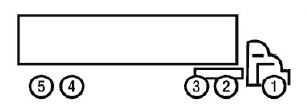Figure 3

Figure 3 shows the most common vehicle checked for compliance with weight limit requirements. Although the Bridge Formula applies to each combination of two or more axles, experience shows that axle combinations 1 through 3, 1 through 5, and 2 through 5 are critical and must be checked. If these combinations are found to be satisfactory, then all of the others on this type of vehicle normally will be satisfactory.

The vehicle with weights and axle dimensions shown in Figure 4 is used to illustrate a Bridge Formula check.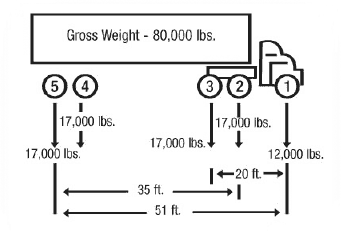Figure 4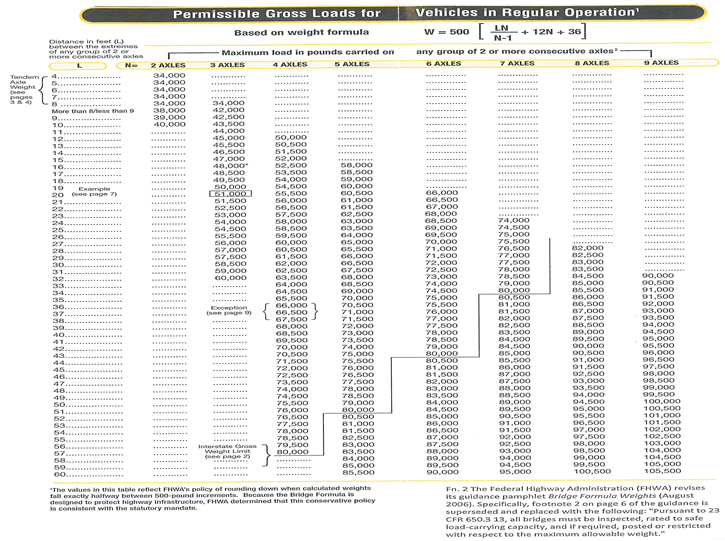Permissible Gross Loads for Vehicles in Regular Operation1

Before checking for compliance with the Bridge Formula, a vehicle's single-axle, tandem-axle, and gross weight should be checked. Here the single axle (number 1) does not exceed 20,000 pounds, tandems 2-3 and 4-5 do not exceed 34,000 pounds each, and the gross weight does not exceed 80,000 pounds. Thus, these preliminary requirements are satisfied. The first Bridge Formula combination is checked as follows: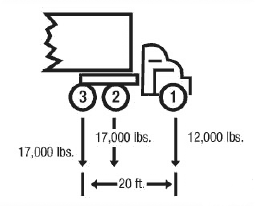Figure 5

### Check axles 1 through 3 (Figure 5)

Actual weight = 12,000 + 17,000 + 17,000 = 46,000 pounds.

N = 3 axles

L = 20 feet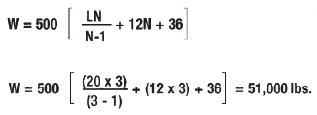Maximum weight (W) = 51,000 pounds, which is more than the actual weight of 46,000 pounds. Thus, the Bridge Formula requirement is satisfied.

### Example From the Bridge Table (pages 5 & 6)

This same number (51,000 pounds) could have been obtained from the Bridge Table by reading down the left side to L = 20 and across to the right where N = 3.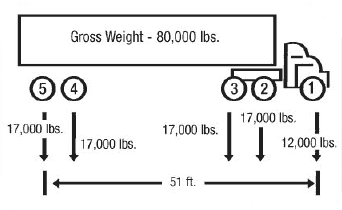Figure 6

### Now check axles 1 through 5 (Figure 6)

Actual weight = 12,000 + 17,000 + 17,000 + 17,000 + 17,000 = 80,000 pounds.

Maximum weight (W) = 80,000 pounds (Bridge Table for "L" of 51 feet and "N" of 5 axles).

Therefore, this axle spacing is satisfactory.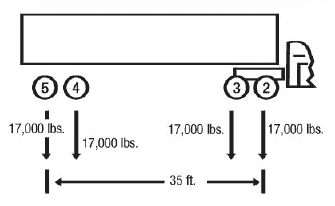Figure 7

### Now check axles 2 through 5 (Figure 7)

Actual weight = 17,000 + 17,000 + 17,000 + 17,000 = 68,000 pounds.

Maximum weight (W) = 65,500 pounds (Bridge Table for "L" of 35 feet and "N" of 4 axles).

This is a violation because the actual weight exceeds the weight allowed by the Bridge Formula. To correct the situation, some load must be removed from the vehicle or the axle spacing (35 feet) must be increased.

## Exception to Formula and Bridge Table

In addition to the grandfather rights notes on page 3, Federal law (23 U.S.C. 127) includes one other exception to the Bridge Formula and the Bridge Table—two consecutive sets of tandem axles may carry 34,000 pounds each if the overall distance between the first and last axles of these tandems is 36 feet or more. For example, a five-axle tractor-semitrailer combination may carry 34,000 pounds both on the tractor tandem (axles 2 and 3) and the trailer tandem (axles 4 and 5), provided axles 2 and 5 are spaced at least 36 feet apart. Without this exception, the Bridge Formula would allow an actual weight of only 66,000 to 67,500 pounds on tandems spaced 36 to 38 feet apart.

## Bridge Formula Application to Single-Unit Trucks

The procedure described above could be used to check any axle combinations, but several closely spaced axles usually produce the most critical situation.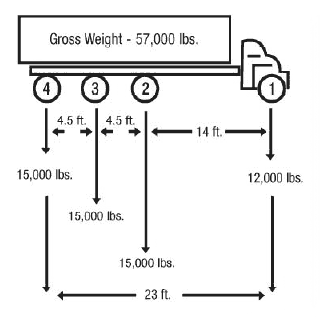Figure 8

The truck shown in Figure 8 satisfies the single-axle weight limit (12,000 pounds are less than 20,000 pounds), the tandem-axle limit (30,000 pounds are less than 34,000 pounds) and the gross-weight limit (57,000 pounds are less than 80,000 pounds). With these restrictions satisfied, a check is done for Bridge Formula requirements, axles 1 through 4.

Actual weight = 12,000 + 15,000 + 15,000 + 15,000 = 57,000 pounds.

Maximum weight (W) = 57,500 pounds (Bridge Table for "L" of 23 feet and "N" of 4 axles).

### Since axles 1 through 4 are satisfactory, check axles 2 through 4:

Actual weight = 15,000 + 15,000 + 15,000 = 45,000 pounds.

Maximum weight (W) = 42,500 pounds (Bridge Table for "L" of 9 feet and "N" of 3 axles.

This is a violation because the actual weight exceeds the weight allowed by the Bridge Formula. The load must either be reduced, axles added, or spacing increased to comply with the Bridge Formula.

## Quality Assurance Statement

The Federal Highway Administration (FHWA) provides high-quality information to serve Government, industry, and the public in a manner that promotes public understanding. Standards and policies are used to ensure and maximize the quality, objectivity, utility, and integrity of its information. FHWA periodically reviews quality issues and adjusts its programs and processes to ensure continuous quality improvement.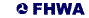United States Department of Transportation - Federal Highway Administration